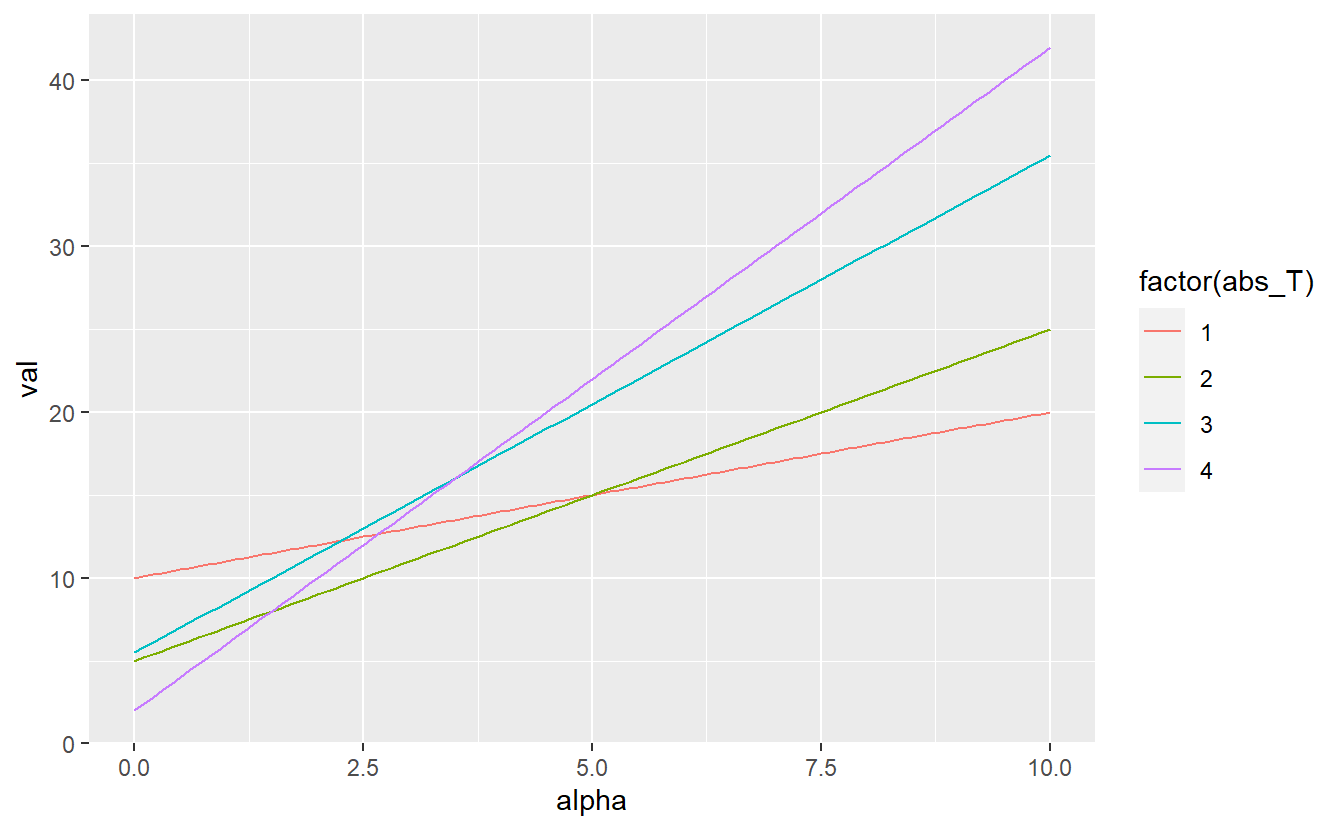# 11 Random Forests

In the last chapter, we used the tidymodels package to build a classification model for the titanic data set from the infamous kaggle competition of the same name. More precisely, we

• split our data for training and testing purposes,
• handled all pre-processing steps by using a recipe,
• used logistic regression to implement our model specification and
• used a workflow to coordinate all of these parts.

Due to this compartmentalization of tasks you may suspect that the previous steps can be easily adapted to accommodate different model specification, e.g. if we want to move away from using a logistic regression. Luckily, this is exactly the case. To change our model, we have to only tweak the model specification and possibly add or remove a few preprocessing steps, e.g. those that were added only for technical reasons like `step_zv()`.

In this chapter, we want to introduce a new model specification, namely random forests. Even without knowing how this model works, we can state that what we need to change compared to our calculations from the last chapter requires us to

• change the ‘standard technical steps’ for logistic regression to those of random forests and
• change the model specification.

This is done as follows. Notice that most of the code is simply copied from the previous chapter.

``````library(tidyverse)
library(tidymodels)
titanic <- read_csv("data/titanic.csv")
titanic <- titanic %>%
mutate(
Survived = factor(
Survived,
levels = c(0,1),
labels = c("Deceased", "Survived")
)
)

############# Data Splitting stays the same
set.seed(123)
titanic_split <- initial_split(titanic, strata = Survived)
titanic_train <- training(titanic_split)
titanic_test <- testing(titanic_split)
set.seed(456)
titanic_folds <- vfold_cv(titanic_train, strata = Survived)

############# Change only technical recipe steps
titanic_rec <- recipe(formula = Survived ~ ., data = titanic_train) %>%
### Our pre-processing from the previous analysis
update_role(PassengerId, new_role = "Id") %>%
step_mutate(Cabin = if_else(is.na(Cabin), "Missing", "Available")) %>%
step_impute_median(Age) %>%
step_mutate(
title = str_match(Name, ", ([:alpha:]+)\\."),
title = if_else(is.na(title[, 2]), "NA", title[, 2])
) %>%
step_other(title, threshold = 0.02, other = "Other") %>%
step_rm(Ticket, Embarked) %>%
update_role(Name, new_role = "Id") %>%
### Leave only one "technical step"
step_string2factor(all_nominal_predictors())

############# New model specification
############# tune() explained in a sec
titanic_spec <-
rand_forest(mtry = tune(), min_n = tune(), trees = 1000) %>%
set_mode("classification") %>%
set_engine("ranger")

############# Workflow stays the same
titanic_wf <- workflow() %>%
add_recipe(titanic_rec) %>%
add_model(titanic_spec)``````

As you can see from this random forest can be considered as “low maintenance models” as they require only very little technical steps. Further, you may have noticed that the new model specification uses (cryptic) arguments `mtry`, `min_n` and `trees` in conjunction

``````usemodels::use_ranger(Survived ~ ., data = titanic)
#> ranger_recipe <-
#>   recipe(formula = Survived ~ ., data = titanic) %>%
#>   step_string2factor(one_of("Name", "Sex", "Ticket", "Cabin", "Embarked"))
#>
#> ranger_spec <-
#>   rand_forest(mtry = tune(), min_n = tune(), trees = 1000) %>%
#>   set_mode("classification") %>%
#>   set_engine("ranger")
#>
#> ranger_workflow <-
#>   workflow() %>%
#>   add_recipe(ranger_recipe) %>%
#>   add_model(ranger_spec)
#>
#> set.seed(44641)
#> ranger_tune <-
#>   tune_grid(ranger_workflow, resamples = stop("add your rsample object"), grid = stop("add number of candidate points"))``````
``````library(tidyverse)

expand_grid(
SSR = c(2, 5, 5.5, 10),
alpha = seq(0, 10, 0.1)
) %>%
mutate(abs_T = case_when(
SSR == 2 ~ 4,
SSR == 5.5 ~ 3,
SSR == 5 ~ 2,
SSR == 10 ~ 1,
)) %>%
mutate(val = SSR + alpha * abs_T) %>%
ggplot(aes(alpha, val, col = factor(abs_T))) +
geom_line()``````